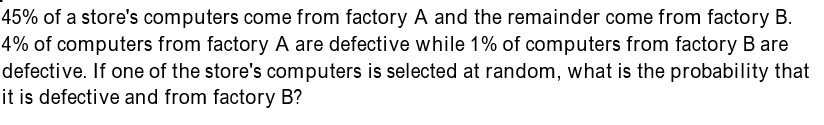# 45% of a store's computers come from factory A and the remainder come from factory B.4% of computers from factory A are defective while 1% of computers from factory B aredefective. If one of the store's computers is selected at random, what is the probability thatit is defective and from factory B?

Question
87 viewshelp_outlineImage Transcriptionclose45% of a store's computers come from factory A and the remainder come from factory B. 4% of computers from factory A are defective while 1% of computers from factory B are defective. If one of the store's computers is selected at random, what is the probability that it is defective and from factory B? fullscreen
check_circle

Step 1

Introduction:

Let A be the event that a randomly selected computer in the store is from factory A; so, AC denotes the event that a randomly selected computer in the store is from factory B.

Given that, P (A) = 0.45; P (AC) = 0.55 (= 1 &...

### Want to see the full answer?

See Solution

#### Want to see this answer and more?

Solutions are written by subject experts who are available 24/7. Questions are typically answered within 1 hour.*

See Solution
*Response times may vary by subject and question.
Tagged in

### Basic Probability